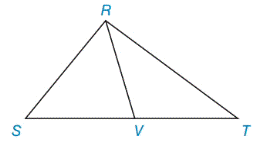Chapter 8.1, Problem 30EElementary Geometry For College St...

7th Edition
Alexander + 2 others
ISBN: 9781337614085

Solutions

Chapter
SectionElementary Geometry For College St...

7th Edition
Alexander + 2 others
ISBN: 9781337614085
Textbook Problem

The following problem is based on this theorem: “A median of a triangle separates it into two triangles of equal area.”In the figure, △ R S T has median R V ¯ .a) Explain why A R S V = A R V T .b) If A R S T = 40.8  cm 2 , find A R S V .To determine

a)

To explain:

ARSV=ARVT. If ΔRST has median RV¯

Explanation

Given,

In figure, ΔRST has median RV¯

Here, RV¯ separates the triangles RST into two triangles ΔRSV and ΔRVT.

Since, RV¯ is the median of ST¯

Let b be the length of base of ΔRST

(i.e.) ST¯=b and

Then, SV¯=b2 and VT¯=b2

(i.e.) SV¯=VT¯=b2

In both triangle of ΔRSV and ΔRVT, height is same

To determine

b)

To find:

ARSV if ARST=40.8 cm2.

Still sussing out bartleby?

Check out a sample textbook solution.

See a sample solution

The Solution to Your Study Problems

Bartleby provides explanations to thousands of textbook problems written by our experts, many with advanced degrees!

Get Started

Find more solutions based on key concepts